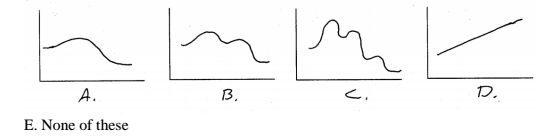Problem: Which of the following energy diagrams is of a reaction with one transition state?

FREE Expert Solution
90% (382 ratings)
Problem Details

Which of the following energy diagrams is of a reaction with one transition state?What scientific concept do you need to know in order to solve this problem?

Our tutors have indicated that to solve this problem you will need to apply the Energy Diagram concept. You can view video lessons to learn Energy Diagram Or if you need more Energy Diagram practice, you can also practice Energy Diagram practice problems .

What is the difficulty of this problem?

Our tutors rated the difficulty of Which of the following energy diagrams is of a reaction with... as medium difficulty.

How long does this problem take to solve?

Our expert Organic tutor, Jonathan took 4 minutes to solve this problem. You can follow their steps in the video explanation above.

What professor is this problem relevant for?

Based on our data, we think this problem is relevant for Professor Daoudi's class at UCF.